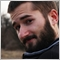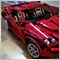# I cannot get the MA to change to color25

```#property copyright "Dusan Fajler"

//--- indicator settings
#property indicator_chart_window
#property indicator_buffers 2
#property indicator_plots   1
#property indicator_type1   DRAW_LINE
#property indicator_color1  clrBlue, clrYellow, clrGreen, clrRed, clrPurple, clrWhite, clrOrange
//--- input parameters
input int            InpMAPeriod=13;         // Period
input int            InpMAShift=0;           // Shift
input ENUM_MA_METHOD InpMAMethod=MODE_SMMA;  // Method

//--- indicator buffers
double ExtLineBuffer[];
double ColorBuffer[];
//+------------------------------------------------------------------+
//|   simple moving average                                          |
//+------------------------------------------------------------------+
void CalculateSimpleMA(int rates_total,int prev_calculated,int begin,const double &price[])
{
int i,limit;
//--- first calculation or number of bars was changed
if(prev_calculated==0)// first calculation
{
limit=InpMAPeriod+begin;
//--- set empty value for first limit bars
for(i=0;i<limit-1;i++) ExtLineBuffer[i]=0.0;
//--- calculate first visible value
double firstValue=0;
for(i=begin;i<limit;i++)
firstValue+=price[i];
firstValue/=InpMAPeriod;
ExtLineBuffer[limit-1]=firstValue;
}
else limit=prev_calculated-1;

//--- main loop
for(i=limit;i<rates_total && !IsStopped();i++)
ExtLineBuffer[i]=ExtLineBuffer[i-1]+(price[i]-price[i-InpMAPeriod])/InpMAPeriod;
//---
}
//+------------------------------------------------------------------+
//|  exponential moving average                                      |
//+------------------------------------------------------------------+
void CalculateEMA(int rates_total,int prev_calculated,int begin,const double &price[])
{
int    i,limit;
double SmoothFactor=2.0/(1.0+InpMAPeriod);
//--- first calculation or number of bars was changed
if(prev_calculated==0)
{
limit=InpMAPeriod+begin;
ExtLineBuffer[begin]=price[begin];
for(i=begin+1;i<limit;i++)
ExtLineBuffer[i]=price[i]*SmoothFactor+ExtLineBuffer[i-1]*(1.0-SmoothFactor);
}
else limit=prev_calculated-1;
//--- main loop
for(i=limit;i<rates_total && !IsStopped();i++)
ExtLineBuffer[i]=price[i]*SmoothFactor+ExtLineBuffer[i-1]*(1.0-SmoothFactor);
//---

}
//+------------------------------------------------------------------+
//|  linear weighted moving average                                  |
//+------------------------------------------------------------------+
void CalculateLWMA(int rates_total,int prev_calculated,int begin,const double &price[])
{
int        i,limit;
static int weightsum;
double     sum;
//--- first calculation or number of bars was changed
if(prev_calculated==0)
{
weightsum=0;
limit=InpMAPeriod+begin;
//--- set empty value for first limit bars
for(i=0;i<limit;i++) ExtLineBuffer[i]=0.0;
//--- calculate first visible value
double firstValue=0;
for(i=begin;i<limit;i++)
{
int k=i-begin+1;
weightsum+=k;
firstValue+=k*price[i];
}
firstValue/=(double)weightsum;
ExtLineBuffer[limit-1]=firstValue;
}
else limit=prev_calculated-1;
//--- main loop
for(i=limit;i<rates_total && !IsStopped();i++)
{
sum=0;
for(int j=0;j<InpMAPeriod;j++) sum+=(InpMAPeriod-j)*price[i-j];
ExtLineBuffer[i]=sum/weightsum;
}
//---
}
//+------------------------------------------------------------------+
//|  smoothed moving average                                         |
//+------------------------------------------------------------------+
void CalculateSmoothedMA(int rates_total,int prev_calculated,int begin,const double &price[])
{
int i,limit;
//--- first calculation or number of bars was changed
if(prev_calculated==0)
{
limit=InpMAPeriod+begin;
//--- set empty value for first limit bars
for(i=0;i<limit-1;i++) ExtLineBuffer[i]=0.0;
//--- calculate first visible value
double firstValue=0;
for(i=begin;i<limit;i++)
firstValue+=price[i];
firstValue/=InpMAPeriod;
ExtLineBuffer[limit-1]=firstValue;
}
else limit=prev_calculated-1;
//--- main loop
for(i=limit;i<rates_total && !IsStopped();i++)
ExtLineBuffer[i]=(ExtLineBuffer[i-1]*(InpMAPeriod-1)+price[i])/InpMAPeriod;
//---
}
//+------------------------------------------------------------------+
//| Custom indicator initialization function                         |
//+------------------------------------------------------------------+
void OnInit()
{
//--- indicator buffers mapping
SetIndexBuffer(0,ExtLineBuffer,INDICATOR_DATA);
//--- set accuracy
IndicatorSetInteger(INDICATOR_DIGITS,_Digits+1);
//--- sets first bar from what index will be drawn
PlotIndexSetInteger(0,PLOT_DRAW_BEGIN,InpMAPeriod);
//---- line shifts when drawing
PlotIndexSetInteger(0,PLOT_SHIFT,InpMAShift);

//--- name for DataWindow
string short_name="unknown ma";
switch(InpMAMethod)
{
case MODE_EMA :  short_name="EMA";  break;
case MODE_LWMA : short_name="LWMA"; break;
case MODE_SMA :  short_name="SMA";  break;
case MODE_SMMA : short_name="SMMA"; break;
}
IndicatorSetString(INDICATOR_SHORTNAME,short_name+"("+string(InpMAPeriod)+")");
//---- sets drawing line empty value--
PlotIndexSetDouble(0,PLOT_EMPTY_VALUE,0.0);
//---- initialization done

SetIndexBuffer(1, ColorBuffer, INDICATOR_COLOR_INDEX);

PlotIndexSetInteger(0, PLOT_COLOR_INDEXES, 3); //Specify the number of color indexes, used in the graphic plot

PlotIndexSetInteger(0, PLOT_LINE_COLOR, 0, clrWhite);  // 0 Index -> White
PlotIndexSetInteger(0, PLOT_LINE_COLOR, 1, clrGreen);  // 1 Index -> Green
PlotIndexSetInteger(0, PLOT_LINE_COLOR, 2, clrOrange); // 2 Index -> Orange

}
//+------------------------------------------------------------------+
//|  Moving Average                                                  |
//+------------------------------------------------------------------+
int OnCalculate(const int rates_total,
const int prev_calculated,
const int begin,
const double &price[])
{
//--- check for bars count
if(rates_total<InpMAPeriod-1+begin)
return(0);// not enough bars for calculation
//--- first calculation or number of bars was changed
if(prev_calculated==0)
{
ArrayInitialize(ExtLineBuffer,0);
ArrayInitialize(ColorBuffer, 0);
}

//--- sets first bar from what index will be draw
PlotIndexSetInteger(0,PLOT_DRAW_BEGIN,InpMAPeriod-1+begin);

//--- calculation
switch(InpMAMethod)
{
case MODE_EMA:  CalculateEMA(rates_total,prev_calculated,begin,price);        break;
case MODE_LWMA: CalculateLWMA(rates_total,prev_calculated,begin,price);       break;
case MODE_SMMA: CalculateSmoothedMA(rates_total,prev_calculated,begin,price); break;
case MODE_SMA:  CalculateSimpleMA(rates_total,prev_calculated,begin,price);   break;
}

ColorBuffer[rates_total-1] = 1;

//--- return value of prev_calculated for next call

return(rates_total);
}
//+------------------------------------------------------------------+
```

I cannot see what am Idoing wrong750

Dusan Fajler:

I cannot see what am Idoing wrong

Here's the main culprit:

```#property indicator_type1   DRAW_LINE
```

And here's a quick and dirty way to test:

```     int iLimit = MathMin(rates_total-1,rates_total-prev_calculated);
for (int i=iLimit; i>=0; i--)
ColorBuffer[i] = i%3;
```25

Always white, same as before750

Dusan Fajler:
Oops! I thought I added 'color'... 😂 Should be :
`#property indicator_type1   DRAW_COLOR_LINE`25

Seng Joo Thio:
Oops! I thought I added 'color'... 😂 Should be :

It works! Thanks a lot!